# 5.4 Probes

In addition to controllers and monitors, applications can also attach streams of data, known as probes, to input and output values associated with the simulation. Probes derive from the same base class ModelAgentBase as controllers and monitors, but differ in that

1. 1.

They are associated with an explicit time interval during which they are applied;

2. 2.

They can have an attached file for supplying input data or recording output data;

3. 3.

They are displayable in the ArtiSynth timeline panel.

A probe is applied (by calling its apply() method) only for time steps that fall within its time interval. This interval can be set and queried using the following methods:

setStartTime (double t0);
setStopTime (double t1);
setInterval (double t0, double t1);
double getStartTime();
double getStopTime();

The probe’s attached file can be set and queried using:

setAttachedFileName (String fileName);
String getAttachedFileName ();

where fileName is a string giving the file’s path name.

Details about the timeline display can be found in the section “The Timeline” in the ArtiSynth User Interface Guide.

There are two types of probe: input probes, which are applied at the beginning of each simulation step before the controllers, and output probes, which are applied at the end of the step after the monitors.

While applications are free to construct any type of probe by subclassing either InputProbe or OutputProbe, most applications utilize either NumericInputProbe or NumericOutputProbe, which explicitly implement streams of numeric data which are connected to properties of various model components. The remainder of this section will focus on numeric probes.

As with controllers and monitors, probes also implement a isActive() method that indicates whether or not the probe is active. Probes that are not active are not invoked. Probes also provide a setActive() method to control this setting, and export it as the property active. This allows probe activity to be controlled at run time.

To enable or disable a probe at run time, locate it in the navigation panel (under the RootModel’s inputProbes or outputProbes list), chose Edit properties ... from the right-click context menu, and set the active property as desired.
Probes can also be enabled or disabled in the timeline, by either selecting the probe and invoking activate or deactivate from the right-click context menu, or by clicking the track mute button (which activates or deactivates all probes on that track).

## 5.4.1 Numeric probe structure

Numeric probes are associated with:

• A vector of temporally-interpolated numeric data;

• One or more properties to which the probe is bound and which are either set by the numeric data (input probes), or used to set the numeric data (output probes).

The numeric data is implemented internally by a NumericList, which stores the data as a series of vector-valued knot points at prescribed times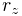and then interpolates the data for an arbitrary time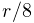using an interpolation scheme provided by Interpolation.

Some of the numeric probe methods associated with the interpolated data include:

int getVsize();                      // returns the size of the data vector
setInterpolationOrder (Order order); // sets the interpolation scheme
Order getInterpolationOrder();       // returns the interpolation scheme
VectorNd getData (double t);         // interpolates data for time t
NumericList getNumericList();        // returns the underlying NumericList

Interpolation schemes are described by the enumerated type Interpolation.Order and presently include:

Step

Values at timeare set to the values of the closest knot point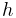such that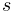.

Linear

Values at timeare set by linear interpolation of the knot points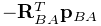such that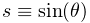.

Parabolic

Values at timeare set by quadratic interpolation of the knots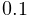such that.

Cubic

Values at timeare set by cubic Catmull interpolation of the knots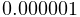such that.

Each property bound to a numeric probe must have a value that can be mapped onto a scalar or vector value. Such properties are know as numeric properties, and whether or not a value is numeric can be tested usingNumericConverter.isNumeric(value).

By default, the total number of scalar and vector values associated with all the properties should equal the size of the interpolated vector (as returned by getVsize()). However, it is possible to establish more complex mappings between the property values and the interpolated vector. These mappings are beyond the scope of this document, but are discussed in the sections “General input probes” and “General output probes” of the ArtiSynth User Interface Guide.

## 5.4.2 Creating probes in code

This section discusses how to create numeric probes in code. They can also be created and added to a model graphically, as described in the section “Adding and Editing Numeric Probes” in the ArtiSynth User Interface Guide.

Numeric probes have a number of constructors and methods that make it relatively easy to create instances of them in code. For NumericInputProbe, there is the constructor

NumericInputProbe (ModelComponent c, String propPath, String filePath);

which creates a NumericInputProbe, binds it to a property located relative to the component c by propPath, and then attaches it to the file indicated by filePath and loads data from this file (see Section 5.4.4). The probe’s start and stop times are specified in the file, and its vector size is set to match the size of the scalar or vector value associated with the property.

To create a probe attached to multiple properties, one may use the constructor

NumericInputProbe (ModelComponent c, String propPaths[], String filePath);

which binds the probe to multiple properties specified relative to c by propPaths. The probe’s vector size is set to the sum of the sizes of the scalar or vector values associated with these properties.

For NumericOutputProbe, one may use the constructor

NumericOutputProbe (ModelComponent c, String propPath, String filePath, double sample);

which creates a NumericOutputProbe, binds it to the property propPath located relative to c, and then attaches it to the file indicated by filePath. The argument sample indicates the sample time associated with the probe, in seconds; a value of 0.01 means that data will be added to the probe every 0.01 seconds. If sample is specified as -1, then the sample time will default to the maximum step size associated with the root model.

To create an output probe attached to multiple properties, one may use the constructor

NumericOutputProbe (
ModelComponent c, String propPaths[], String filePath, double sample);

As the simulation proceeds, an output probe will accumulate data, but this data will not be saved to any attached file until the probe’s save() method is called. This can be requested in the GUI for all probes by clicking on the Save button in the timeline toolbar, or for specific probes by selecting them in the navigation panel (or the timeline) and then choosing Save data in the right-click context menu.

Output probes created with the above constructors have a default interval of [0, 1]. A different interval may be set using setInterval(), setStartTime(), or setStopTime().

## 5.4.3 Example: probes connected to SimpleMuscle

A model showing a simple application of probes is defined in

  artisynth.demos.tutorial.SimpleMuscleWithProbes


This extends SimpleMuscle (Section 4.4.2) to add an input probe to move particle p1 along a defined path, along with an output probe to record the velocity of the frame marker. The complete class definition is shown below:

1 package artisynth.demos.tutorial;
2
3 import java.io.IOException;
4 import maspack.matrix.*;
5 import maspack.util.PathFinder;
6
7 import artisynth.core.modelbase.*;
8 import artisynth.core.mechmodels.*;
9 import artisynth.core.probes.*;
10
11 public class SimpleMuscleWithProbes extends SimpleMuscleWithPanel
12 {
13    public void createInputProbe() throws IOException {
14       NumericInputProbe p1probe =
15          new NumericInputProbe (
16             mech, "particles/p1:targetPosition",
17             PathFinder.getSourceRelativePath (this, "simpleMuscleP1Pos.txt"));
18       p1probe.setName("Particle Position");
19       addInputProbe (p1probe);
20    }
21
22    public void createOutputProbe() throws IOException {
23       NumericOutputProbe mkrProbe =
24          new NumericOutputProbe (
25             mech, "frameMarkers/0:velocity",
26             PathFinder.getSourceRelativePath (this, "simpleMuscleMkrVel.txt"),
27             0.01);
28       mkrProbe.setName("FrameMarker Velocity");
29       mkrProbe.setDefaultDisplayRange (-4, 4);
30       mkrProbe.setStopTime (10);
31       addOutputProbe (mkrProbe);
32    }
33
34    public void build (String[] args) throws IOException {
35       super.build (args);
36
37       createInputProbe ();
38       createOutputProbe ();
39       mech.setBounds (-1, 0, -1, 1, 0, 1);
40    }
41
42 }

The input and output probes are added using the custom methods createInputProbe() and createOutputProbe(). At line 14, createInputProbe() creates a new input probe bound to the targetPosition property for the component particles/p1 located relative to the MechModel mech. The same constructor attaches the probe to the filesimpleMuscleP1Pos.txt, which is read to load the probe data. The format of this and other probe data files is described in Section 5.4.4. The method PathFinder.getSourceRelativePath() is used to locate the file relative to the source directory for the application model (see Section 2.6). The probe is then given the name "Particle Position" (line 18) and added to the root model (line 19).

Similarly, createOutputProbe() creates a new output probe which is bound to the velocity property for the component particles/0 located relative to mech, is attached to the file simpleMuscleMkrVel.txt located in the application model source directory, and is assigned a sample time of 0.01 seconds. This probe is then named "FrameMarker Velocity" and added to the root model.

The build() method calls super.build() to create everything required for SimpleMuscle, calls createInputProbe() and createOutputProbe() to add the probes, and adjusts the MechModel viewer bounds to make the resulting probe motion more visible.

To run this example in ArtiSynth, select All demos > tutorial > SimpleMuscleWithProbes from the Models menu. After the model is loaded, the input and output probes should appear on the timeline (Figure 5.3). Expanding the probes should display their numeric contents, with the knot points for the input probe clearly visible. Running the model will cause particle p1 to trace the trajectory specified by the input probe, while the velocity of the marker is recorded in the output probe. Figure 5.4 shows an expanded view of both probes after the simulation has run for about six seconds.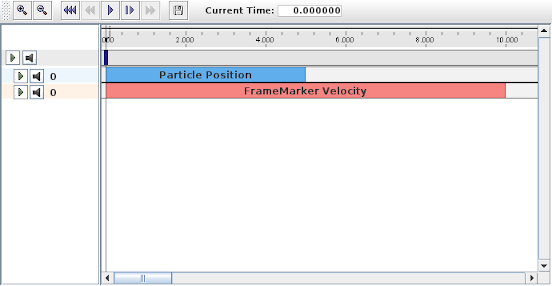Figure 5.3: Timeline view of the probes created by SimpleMuscleWithProbes.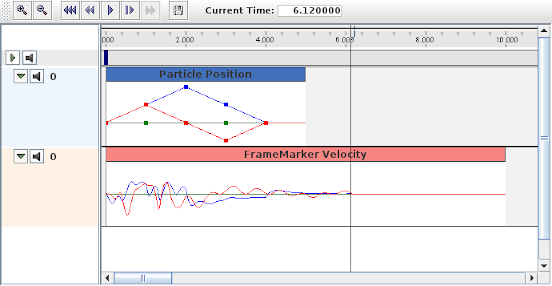Figure 5.4: Expanded view of the probes after SimpleMuscleWithProbes has run for about 6 seconds, showing the data accumulated in the output probe "FrameMarker Velocity".

## 5.4.4 Data file format

The data files associated with numeric probes are ASCII files containing two lines of header information followed by a set of knot points, one per line, defining the numeric data. The time value (relative to the probe’s start time) for each knot point can be specified explicitly at the start of the each line, in which case the file takes the following format:

startTime stopTime scale
interpolation vsize explicit
t0 val00 val01 val02 ...
t1 val10 val11 val12 ...
t0 val20 val21 val22 ...
...

Knot point information begins on line 3, with each line being a sequence of numbers giving the knot’s time followed by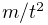values, whereis the vector size of the probe (i.e., the value returned by getVsize()).

Alternatively, time values can be implicitly specified starting at 0 (relative to the probe’s start time) and incrementing by a uniform timeStep, in which case the file assumes a second format:

startTime stopTime scale
interpolation vsize timeStep
val00 val01 val02 ...
val10 val11 val12 ...
val20 val21 val22 ...
...

For both formats, startTime, startTime, and scale are numbers giving the probe’s start and stop time in seconds and scale gives the scale factor (which is typically 1.0). interpolation is a word describing how the data should be interpolated between knot points and is the string value of Interpolation.Order as described in Section 5.4.1 (and which is typically Linear, Parabolic, or Cubic). vsize is an integer giving the probe’s vector size.

The last entry on the second line is either a number specifying a (uniform) time step for the knot points, in which case the file assumes the second format, or the keyword explicit, in which case the file assumes the first format.

As an example, the file used to specify data for the input probe in the example of Section 5.4.3 looks like the following:

0 4.0 1.0
Linear 3 explicit
0.0  0.0 0.0 0.0
1.0  0.5 0.0 0.5
2.0  0.0 0.0 1.0
3.0 -0.5 0.0 0.5
4.0  0.0 0.0 0.

Since the data is uniformly spaced beginning at 0, it would also be possible to specify this using the second file format:

0 4.0 1.0
Linear 3 1.0
0.0 0.0 0.0
0.5 0.0 0.5
0.0 0.0 1.0
-0.5 0.0 0.5
0.0 0.0 0.

## 5.4.5 Adding probe data in-line

It is also possible to specify input probe data directly in code, instead of reading it from a file. For this, one would use the constructor

NumericInputProbe (ModelComponent c, String propPath, double t0, double t1);

which creates a NumericInputProbe with the specified property and with start and stop times indicated by t0 and t1. Data can then be added to this probe using the method

addData (double[] data, double timeStep);

where data is an array of knot point data. This contains the same knot point information as provided by a file (Section 5.4.4), arranged in row-major order. Times values for the knots are either implicitly specified, starting at 0 (relative to the probe’s start time) and increasing uniformly by the amount specified by timeStep, or are explicitly specified at the beginning of each knot if timeStep is set to the built-in constant NumericInputProbe.EXPLICIT_TIME. The size of the data array should then be either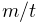(implicit time values) or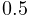(explicit time values), whereis the probe’s vector size and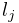is the number of knots.

As an example, the data for the input probe in Section 5.4.3 could have been specified using the following code:

NumericInputProbe p1probe =
new NumericInputProbe (
mech, "particles/p1:targetPosition", 0, 5);
p1probe.addData (
new double[] {
0.0,  0.0, 0.0, 0.0,
1.0,  0.5, 0.0, 0.5,
2.0,  0.0, 0.0, 1.0,
3.0, -0.5, 0.0, 0.5,
4.0,  0.0, 0.0, 0.0 },
NumericInputProbe.EXPLICIT_TIME);

When specifying data in code, the interpolation defaults to Linear unless explicitly specified usingsetInterpolationOrder(), as in, for example:

probe.setInterpolationOrder (Order.Cubic);

## 5.4.6 Numeric monitor probes

In some cases, it may be useful for an application to deploy an output probe in which the data, instead of being collected from various component properties, is generated by a function within the probe itself. This ability is provided by a NumericMonitorProbe, which generates data using its generateData(vec,t,trel) method. This evaluates a vector-valued function of time at either the absolute time t or the probe-relative time trel and stores the result in the vector vec, whose size equals the vector size of the probe (as returned by getVsize()). The probe-relative time trel is determined by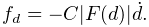(5.1)

where tstart and scale are the probe’s start time and scale factors as returned by getStartTime() and getScale().

As described further below, applications have several ways to control how a NumericMonitorProbe creates data:

The application is free to generate data in any desired way, and so in this sense a NumericMonitorProbe can be used similarly to a Monitor, with one of the main differences being that the data generated by a NumericMonitorProbe can be automatically displayed in the ArtiSynth GUI or written to a file.

The DataFunction interface declares an eval() method,

   void eval (VectorNd vec, double t, double trel)


that for NumericMonitorProbes evaluates a vector-valued function of time, where the arguments take the same role as for the monitor’s generateData() method. Applications can declare an appropriate DataFunction and set or query it within the probe using the methods

void setDataFunction (DataFunction func);
DataFunction getDataFunction();

The default implementation generateData() checks to see if a data function has been specified, and if so, uses that to generate the probe data. Otherwise, if the probe’s data function is null, the data is simply set to zero.

To create a NumericMonitorProbe using a supplied DataFunction, an application will create a generic probe instance, using one of its constructors such as

NumericMonitorProbe (vsize, fileName, startTime, stopTime, interval);

and then define and instantiate a DataFunction and pass it to the probe using setDataFunction(). It is not necessary to supply a file name (i.e., fileName can be null), but if one is provided, then the probe’s data can be saved to that file.

A complete example of this is defined in

  artisynth.demos.tutorial.SinCosMonitorProbe


the listing for which is:

1 package artisynth.demos.tutorial;
2
3 import maspack.matrix.*;
4 import maspack.util.Clonable;
5
6 import artisynth.core.workspace.RootModel;
7 import artisynth.core.probes.NumericMonitorProbe;
8 import artisynth.core.probes.DataFunction;
9
10 /**
11  * Simple demo using a NumericMonitorProbe to generate sine and cosine waves.
12  */
13 public class SinCosMonitorProbe extends RootModel {
14
15    // Define the DataFunction that generates a sine and a cosine wave
16    class SinCosFunction implements DataFunction, Clonable {
17
18       public void eval (VectorNd vec, double t, double trel) {
19          // vec should have size == 2, one for each wave
20          vec.set (0, Math.sin (t));
21          vec.set (1, Math.cos (t));
22       }
23
24       public Object clone() throws CloneNotSupportedException {
25          return (SinCosFunction)super.clone();
26       }
27    }
28
29    public void build (String[] args) {
30
31       // Create a NumericMonitorProbe with size 2, file name "sinCos.dat", start
32       // time 0, stop time 10, and a sample interval of 0.01 seconds:
33       NumericMonitorProbe sinCosProbe =
34          new NumericMonitorProbe (/*vsize=*/2, "sinCos.dat", 0, 10, 0.01);
35
36       // then set the data function:
37       sinCosProbe.setDataFunction (new SinCosFunction());
38       addOutputProbe (sinCosProbe);
39    }
40 }

In this example, the DataFunction is implemented using the class SinCosFunction, which also implements Clonable and the associated clone() method. This means that the resulting probe will also be duplicatable within the GUI. Alternatively, one could implement SinCosFunction by extending DataFunctionBase, which implements Clonable by default. Probes containing DataFunctions which are not Clonable will not be duplicatable.

When the example is run, the resulting probe output is shown in the timeline image of Figure 5.5.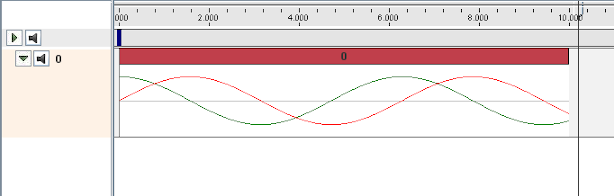Figure 5.5: Output from a NumericMonitorProbe which generates sine and cosine waves.

As an alternative to supplying a DataFunction to a generic NumericMonitorProbe, an application can instead subclass NumericMonitorProbe and override either its generateData(vec,t,trel) or apply(t) methods. As an example of the former, one could create a subclass as follows:

class SinCosProbe extends NumericMonitorProbe {
public SinCosProbe (
String fileName, double startTime, double stopTime, double interval) {
super (2, fileName, startTime, stopTime, interval);
}
public void generateData (VectorNd vec, double t, double trel) {
vec.set (0, Math.sin (t));
vec.set (1, Math.cos (t));
}
}

Note that when subclassing, one must also create constructor(s) for that subclass. Also, NumericMonitorProbes which don’t have a DataFunction set are considered to be clonable by default, which means that the clone() method may also need to be overridden if cloning requires any special handling.

## 5.4.7 Numeric control probes

In other cases, it may be useful for an application to deploy an input probe which takes numeric data, and instead of using it to modify various component properties, instead calls an internal method to directly modify the simulation in any way desired. This ability is provided by a NumericControlProbe, which applies its numeric data using its applyData(vec,t,trel) method. This receives the numeric input data via the vector vec and uses it to modify the simulation for either the absolute time t or probe-relative time trel. The size of vec equals the vector size of the probe (as returned by getVsize()), and the probe-relative time trel is determined as described in Section 5.4.6.

A NumericControlProbe is the Controller equivalent of a NumericMonitorProbe, as described in Section 5.4.6. Applications have several ways to control how they apply their data:

The application is free to apply data in any desired way, and so in this sense a NumericControlProbe can be used similarly to a Controller, with one of the main differences being that the numeric data used can be automatically displayed in the ArtiSynth GUI or read from a file.

The DataFunction interface declares an eval() method,

   void eval (VectorNd vec, double t, double trel)


that for NumericControlProbes applies the numeric data, where the arguments take the same role as for the monitor’s applyData() method. Applications can declare an appropriate DataFunction and set or query it within the probe using the methods

void setDataFunction (DataFunction func);
DataFunction getDataFunction();

The default implementation applyData() checks to see if a data function has been specified, and if so, uses that to apply the probe data. Otherwise, if the probe’s data function is null, the data is simply ignored and the probe does nothing.

To create a NumericControlProbe using a supplied DataFunction, an application will create a generic probe instance, using one of its constructors such as

NumericControlProbe (vsize, data, startTime, stopTime, timeStep);
NumericControlProbe (fileName);

and then define and instantiate a DataFunction and pass it to the probe using setDataFunction(). The latter constructor creates the probe and reads in both the data and timing information from the specified file.

A complete example of this is defined in

  artisynth.demos.tutorial.SpinControlProbe


the listing for which is:

1 package artisynth.demos.tutorial;
2
3 import maspack.matrix.RigidTransform3d;
4 import maspack.matrix.VectorNd;
5 import maspack.util.Clonable;
6 import maspack.interpolation.Interpolation;
7
8 import artisynth.core.mechmodels.MechModel;
9 import artisynth.core.mechmodels.RigidBody;
10 import artisynth.core.mechmodels.Frame;
11 import artisynth.core.workspace.RootModel;
12 import artisynth.core.probes.NumericControlProbe;
13 import artisynth.core.probes.DataFunction;
14
15 /**
16  * Simple demo using a NumericControlProbe to spin a Frame about the z
17  * axis.
18  */
19 public class SpinControlProbe extends RootModel {
20
21    // Define the DataFunction that spins the body
22    class SpinFunction implements DataFunction, Clonable {
23
24       Frame myFrame;
25       RigidTransform3d myTFW0; // initial frame to world transform
26
27       SpinFunction (Frame frame) {
28          myFrame = frame;
29          myTFW0 = new RigidTransform3d (frame.getPose());
30       }
31
32       public void eval (VectorNd vec, double t, double trel) {
33          // vec should have size == 1, giving the current spin angle
34          double ang = Math.toRadians(vec.get(0));
35          RigidTransform3d TFW = new RigidTransform3d();
36          TFW.R.mulRpy (ang, 0, 0);
37          myFrame.setPose (TFW);
38       }
39
40       public Object clone() throws CloneNotSupportedException {
41          return super.clone();
42       }
43    }
44
45    public void build (String[] args) {
46
47       MechModel mech = new MechModel ("mech");
48       addModel (mech);
49
50       // Create a parametrically controlled rigid body to spin:
51       RigidBody body = RigidBody.createBox ("box", 1.0, 1.0, 0.5, 1000.0);
52       mech.addRigidBody (body);
53       body.setDynamic (false);
54
55       // Create a NumericControlProbe with size 1, initial spin data
56       // with time step 2.0, start time 0, and stop time 8.
57       NumericControlProbe spinProbe =
58          new NumericControlProbe (
59             /*vsize=*/1,
60             new double[] { 0.0, 90.0, 0.0, -90.0, 0.0 },
61             2.0, 0.0, 8.0);
62       // set cubic interpolation for a smoother result
63       spinProbe.setInterpolationOrder (Interpolation.Order.Cubic);
64       // then set the data function:
65       spinProbe.setDataFunction (new SpinFunction(body));
66       addInputProbe (spinProbe);
67    }
68 }

This example creates a simple box and then uses a NumericControlProbe to spin it about the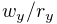axis, using a DataFunction implementation called SpinFunction. A clone method is also implemented to ensure that the probe will be duplicatable in the GUI, as described in Section 5.4.6. A single channel of data is used to control the orientation angle of the box about, as shown in Figure 5.6.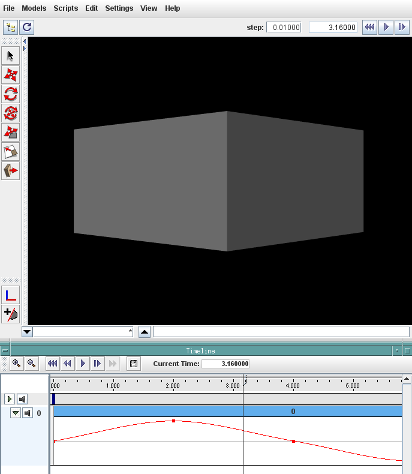Figure 5.6: Screen shot of the SpinControlDemo, showing the numeric data in the timeline.

Alternatively, an application can subclass NumericControlProbe and override either its applyData(vec,t,trel) or apply(t) methods, as described for NumericMonitorProbes (Section 5.4.6).